Like   Tweet   Pin   +1   in

## Elder E-Letter: August 2018

 table.module-2{width:99.81%;padding:0}table div table+table+table div table{width:99.81%;float:none;margin-left:auto;margin-right:auto;padding:0}table div table+table+table div table a{border:0 none;text-decoration:none}table div table+table+table div table img{width:100%!important;border:0 none;text-decoration:none}table div table+table+table div table td{width:100%;padding:0}/* styles */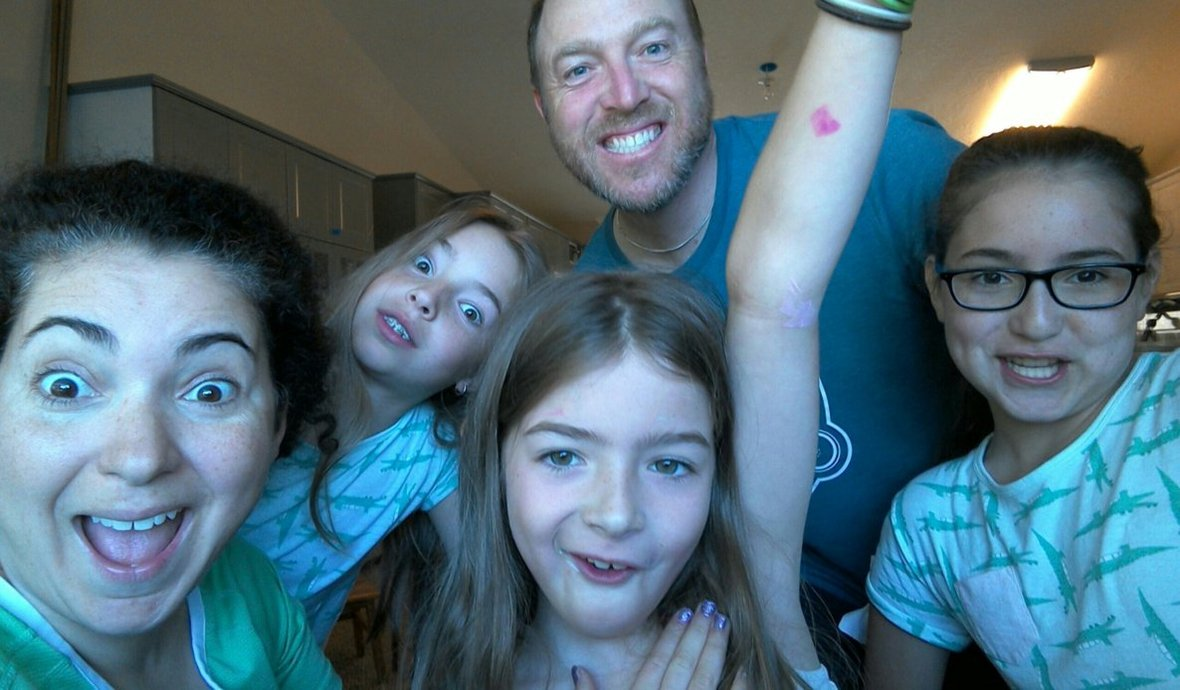Lincoln and his family having funLincoln eating pizza at work in Idaho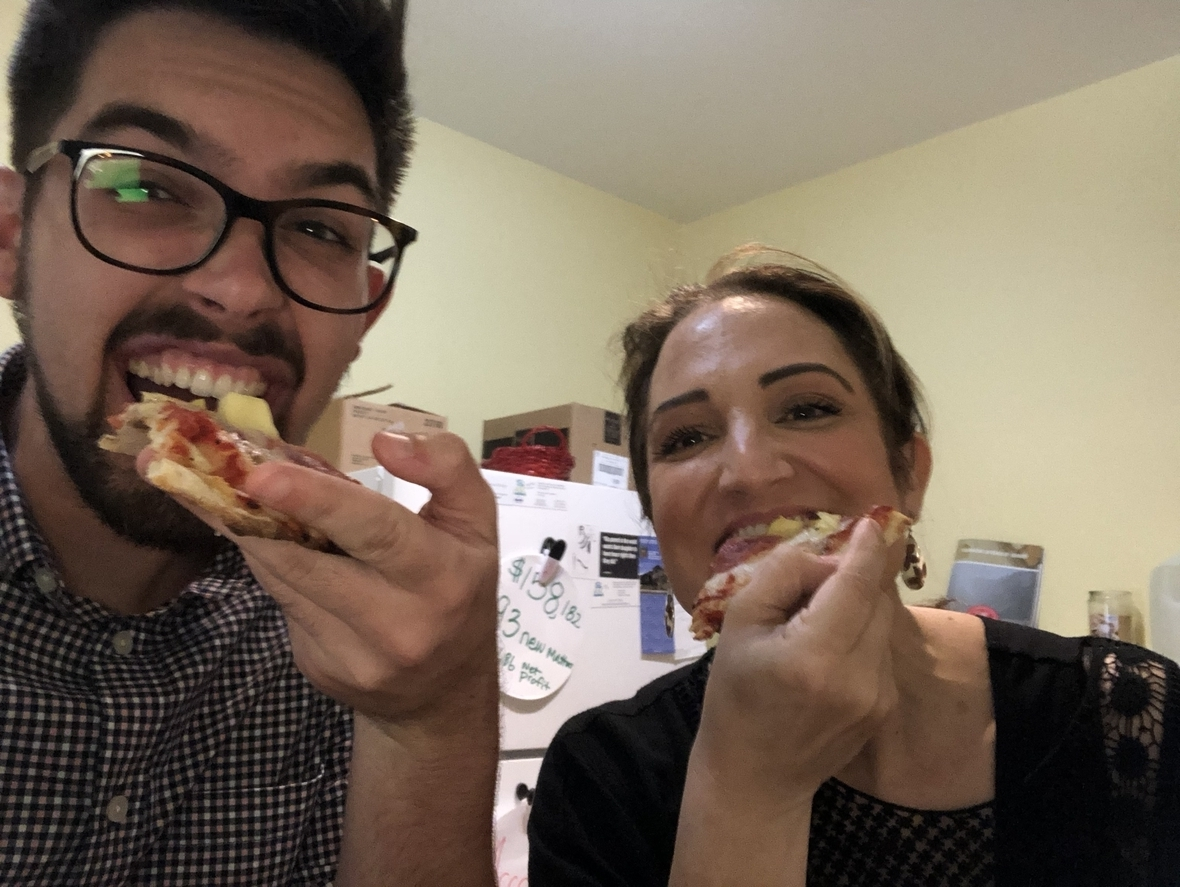Cody and Jody eating pizza while working on the newsletter
 table div table+table+table+table+table+table+table+table div table{width:100%;padding:0}table div table+table+table+table+table+table+table+table div table img{width:96.23%;padding:0;float:none}table div table+table+table+table+table+table+table+table div table td{width:100%;padding:0 1.88% 18px}/* styles */## Come Join The Law Offices of Carol Bertsch for Some Exiciting Fall Events!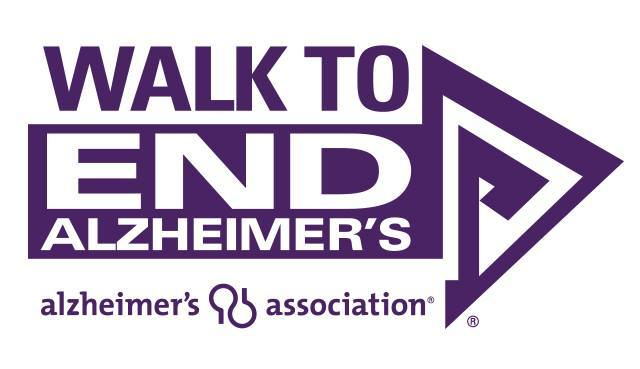/* styles */ 2018 Walk to End Alzheimer's Unite in a movement to reclaim the future for millions. Join our team for the Alzheimer's Association Walk to End Alzheimer's®, the nation's largest event to raise awareness and funds to fight Alzheimer's disease. Together, we can advance research to treat and prevent Alzheimer's, and provide programs and support to improve the lives of millions of affected Americans. Date: October 20, 2018 Registration at 7:00am | Ceremony at 9am | Walk at 9:30am Route Length: 2 miles Location: Palo Alto College 1400 W. Villaret Blvd San Antonio, TX 78224/* styles */ 2018 Buddy Walk The Buddy Walk is an event that celebrates individuals with Down syndrome and increases awareness and inclusion. This one-mile walk is a family event and will include onsite activities such as music, face painting, inflatables, a train, vendor booths, and food trucks. Activities will be from 9:00 am - 1:00 pm. The program will begin at 10:00 am and the walk at 10:30 am. Date: October 20, 2018 Register online Location: Enter Gate B: River City Community Church, 16765 Lookout Road, Selma, TX
 table div table+table+table+table+table+table+table+table+table+table+table+table+table+table div table{width:100%;padding:0}table div table+table+table+table+table+table+table+table+table+table+table+table+table+table div table img{width:96.23%;padding:0;float:none}table div table+table+table+table+table+table+table+table+table+table+table+table+table+table div table td{width:100%;padding:0 1.88% 18px}/* styles */## Caregiver's Corner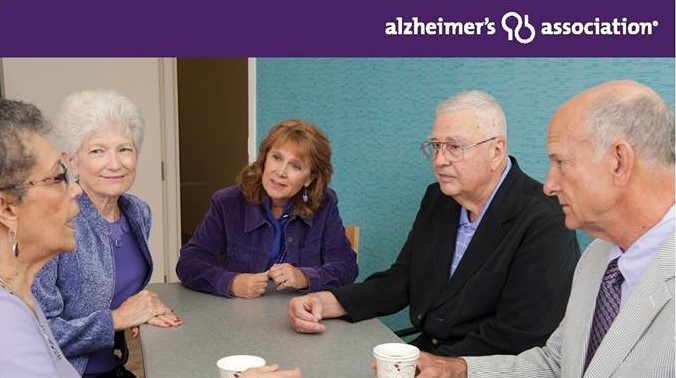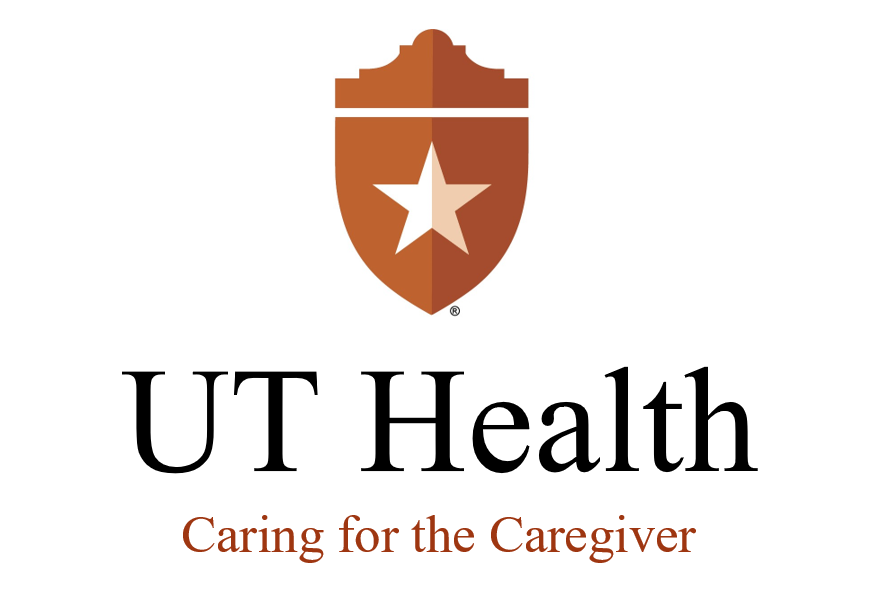## UT Health: Caring for the Caregiver

 /* styles */ Aug. 9 The Essentials of Caregiving: Planning for the Future 2:00-3:00 P.M. Aug. 23 The Essentials of Caregiving: Legal and Financial Planning 2:00-3:00 PM Aug. 30 Cargiver Skills Training Workshop 3:30-6:30 PM

## Alzheimer's Association

 /* styles */ Click here for caregiver resources in Bexar County that are sponsored by the Alzheimer's Association.
 table div table+table+table+table+table+table+table+table+table+table+table+table+table+table+table+table+table+table+table+table+table div table{width:100%;padding:0}table div table+table+table+table+table+table+table+table+table+table+table+table+table+table+table+table+table+table+table+table+table div table img{width:96.23%;padding:0;float:none}table div table+table+table+table+table+table+table+table+table+table+table+table+table+table+table+table+table+table+table+table+table div table td{width:100%;padding:0 1.88% 18px}/* styles */## Senior Service Shoutout/* styles */ Caring Transitions Scott Bleichwehl from Caring Transitions is someone we are proud to recommend. Caring Transitions helps older adults and their families with downsizing, rightsizing, estate sales, and online auctions. After 32 years in the Army, Scott sees his work as an opportunity to continue to serve and give back. “We work with families who need honest, truthful, and trustworthy help. Trust is the number one value that we operate under. We understand how important that is to the clients and their families.” Scott understands the importance of caring for people's personal items they’ve accumulated over the years, and he knows how to liquidate them in appropriate manner. Caring Transitions has an A+ rating with the Better Business Bureau, and all of their employees are background checked. Click here to view their website.
 table div table+table+table+table+table+table+table+table+table+table+table+table+table+table+table+table+table+table+table+table+table+table+table+table+table div table{width:100%;padding:0}table div table+table+table+table+table+table+table+table+table+table+table+table+table+table+table+table+table+table+table+table+table+table+table+table+table div table img{width:96.23%;padding:0;float:none}table div table+table+table+table+table+table+table+table+table+table+table+table+table+table+table+table+table+table+table+table+table+table+table+table+table div table td{width:100%;padding:0 1.88% 18px}/* styles */## Featured Article of the Month/* styles */ The Blog of Bill Gates Why diagnosing Alzheimer’s today is so difficult—and how we can do better "When I announced that I was investing in Alzheimer’s research for the first time last fall, I thought I knew what to expect. I knew I would get to engage more deeply with the brilliant scientists and advocates working to stop Alzheimer’s—and I haven’t been disappointed. The things I’ve seen over the last seven months make me more hopeful than ever." Read More
 table div table+table+table+table+table+table+table+table+table+table+table+table+table+table+table+table+table+table+table+table+table+table+table+table+table+table+table+table+table div table{width:100%;padding:0}table div table+table+table+table+table+table+table+table+table+table+table+table+table+table+table+table+table+table+table+table+table+table+table+table+table+table+table+table+table div table img{width:96.23%;padding:0;float:none}table div table+table+table+table+table+table+table+table+table+table+table+table+table+table+table+table+table+table+table+table+table+table+table+table+table+table+table+table+table div table td{width:100%;padding:0 1.88% 18px}/* styles */## Coming up

 /* styles */ Aug. 7 / Aug. 14 - Carol will lead two classes on First Responder Training for approaching people with Alzheimer's at the San Antonio Police Academy August 9-10 - Carol attends UT Law’s Estate Planning, Guardianship and Elder Law Conference in Galveston, TX. August 14 - Carol talks about Who decides When I Can't - Guardianship and Powers of Attorney at OASIS August 23 - Carol talks about the The Essentials of Caregiving: Legal and Financial Planning for UT Caring for the Caregiver
 table div table+table+table+table+table+table+table+table+table+table+table+table+table+table+table+table+table+table+table+table+table+table+table+table+table+table+table+table+table+table+table+table div table{width:100%;padding:0}table div table+table+table+table+table+table+table+table+table+table+table+table+table+table+table+table+table+table+table+table+table+table+table+table+table+table+table+table+table+table+table+table div table img{width:96.23%;padding:0;float:none}table div table+table+table+table+table+table+table+table+table+table+table+table+table+table+table+table+table+table+table+table+table+table+table+table+table+table+table+table+table+table+table+table div table td{width:100%;padding:0 1.88% 18px}/* styles */## Happenings

 /* styles */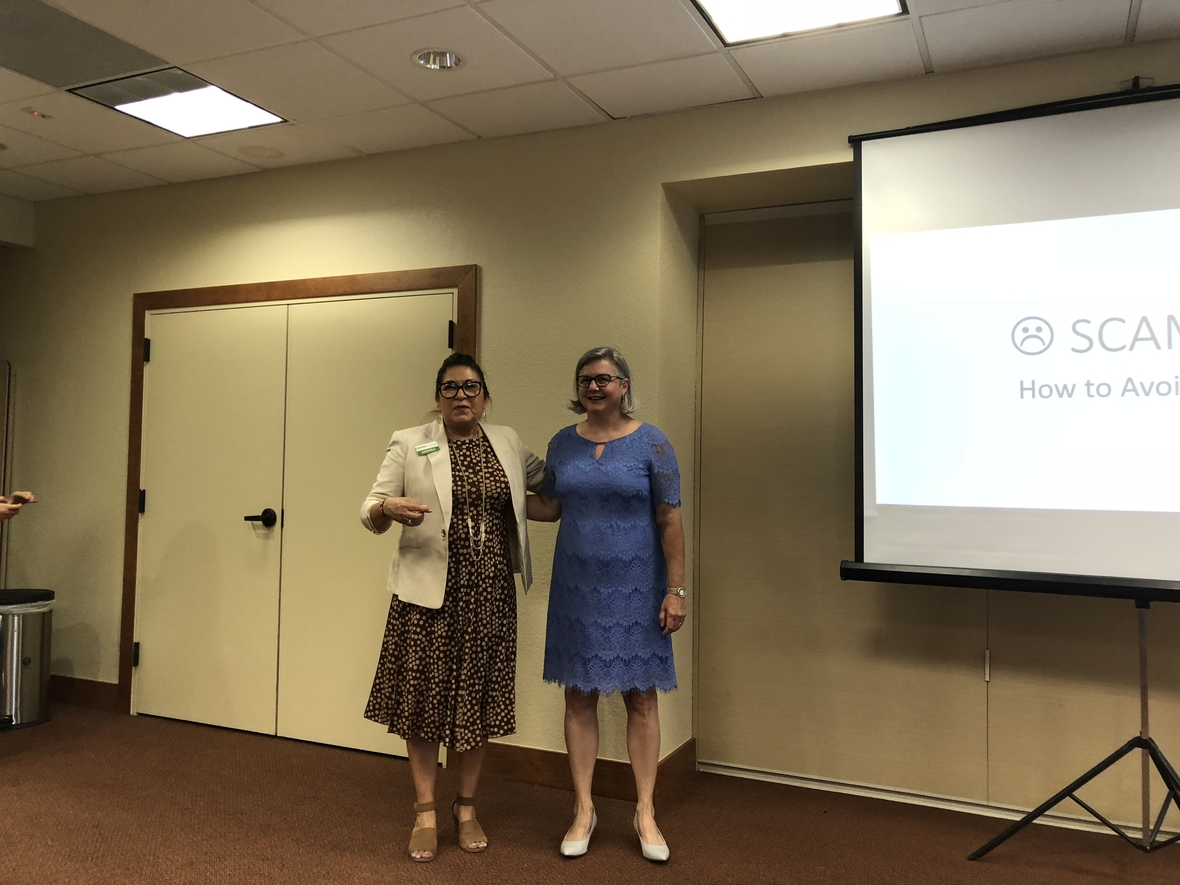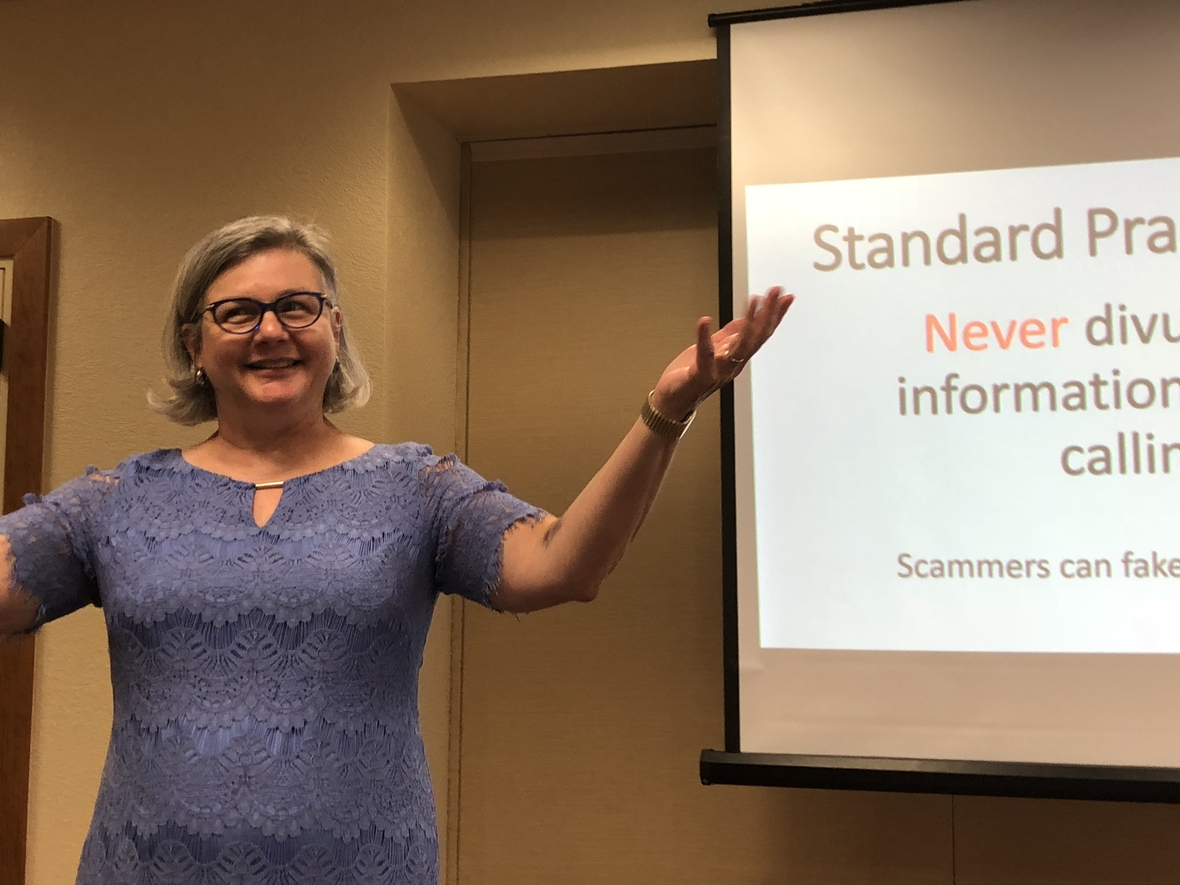/* styles */ July 20 - Carol spoke about senior financial scams at Villa de San Antonio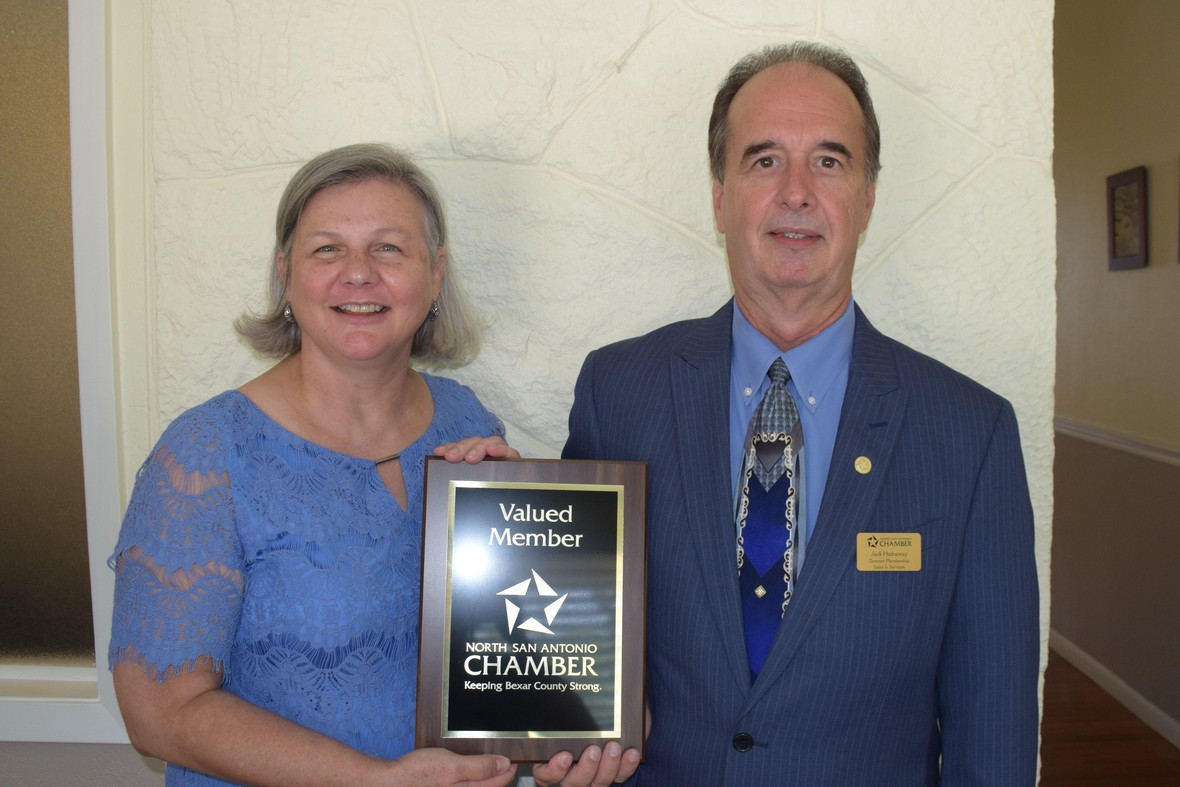/* styles */ July 20 Carol became a member of the North San Antonio Chamber of Commerce. Here's Jack Hathaway presenting her with a plaque.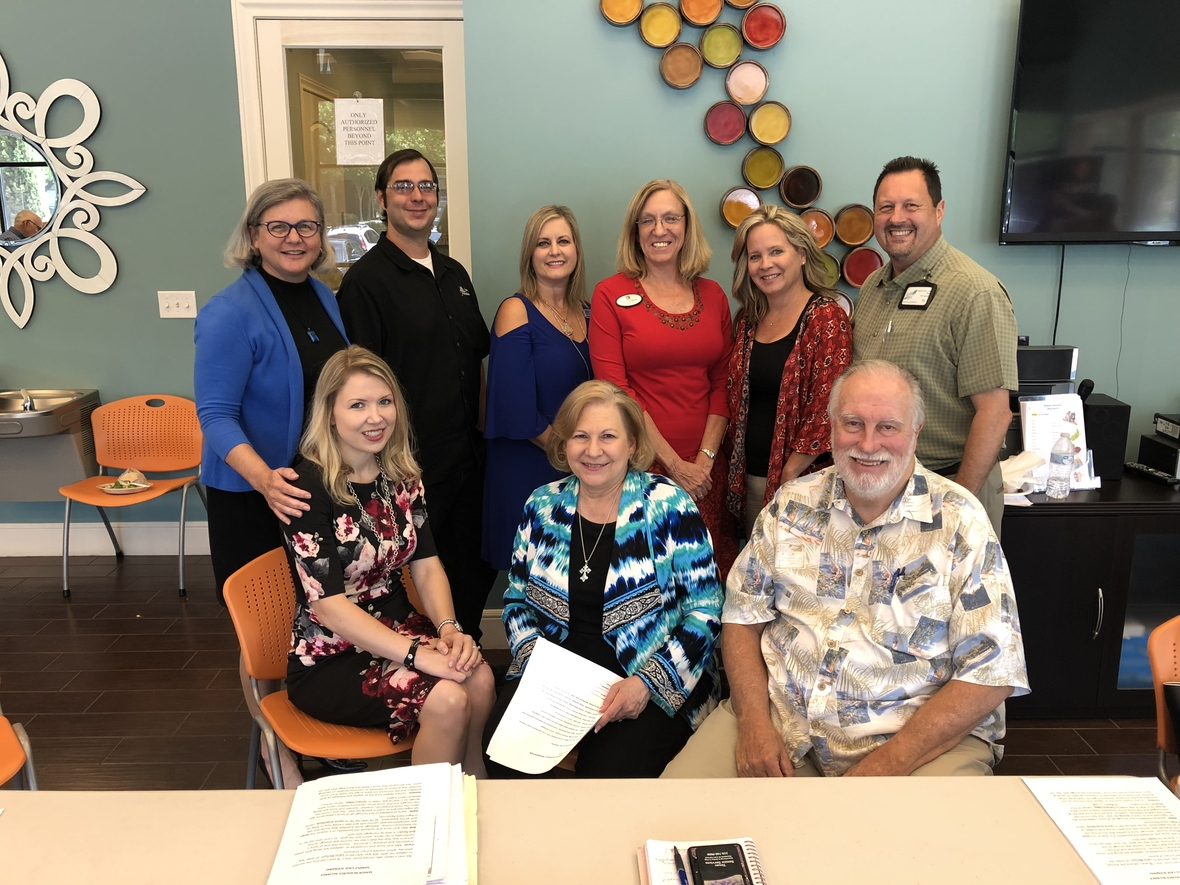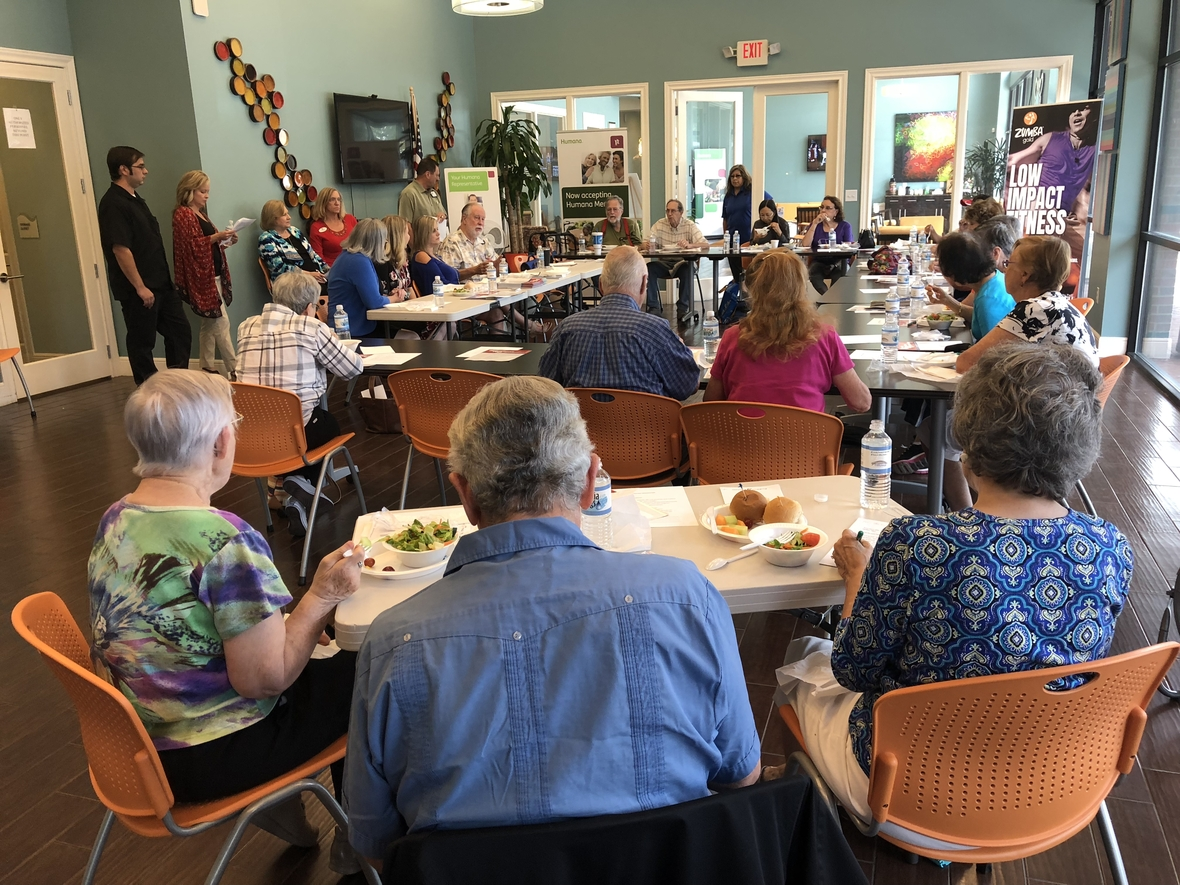/* styles */ July 24 - Carol participated in a pannel discussion about how the Senior Resource Organization can help seniors in the San Antonio community for MCCI Medical Group
 table div table+table+table+table+table+table+table+table+table+table+table+table+table+table+table+table+table+table+table+table+table+table+table+table+table+table+table+table+table+table+table+table+table+table+table+table+table+table+table+table+table div table{width:100%;padding:0}table div table+table+table+table+table+table+table+table+table+table+table+table+table+table+table+table+table+table+table+table+table+table+table+table+table+table+table+table+table+table+table+table+table+table+table+table+table+table+table+table+table div table img{width:96.23%;padding:0;float:none}table div table+table+table+table+table+table+table+table+table+table+table+table+table+table+table+table+table+table+table+table+table+table+table+table+table+table+table+table+table+table+table+table+table+table+table+table+table+table+table+table+table div table td{width:100%;padding:0 1.88% 18px}/* styles */## What’s Going on in Your World?/* styles */ We appreciate your feedback. Do you have a book or a topic you'd like to see featured in our newsletter? Please email your suggestions to jhudspeth@assistingseniors.com. All ideas are welcome.
 table div table+table+table+table+table+table+table+table+table+table+table+table+table+table+table+table+table+table+table+table+table+table+table+table+table+table+table+table+table+table+table+table+table+table+table+table+table+table+table+table+table+table+table+table+table div table{width:100%;padding:0}table div table+table+table+table+table+table+table+table+table+table+table+table+table+table+table+table+table+table+table+table+table+table+table+table+table+table+table+table+table+table+table+table+table+table+table+table+table+table+table+table+table+table+table+table+table div table img{width:96.23%;padding:0;float:none}table div table+table+table+table+table+table+table+table+table+table+table+table+table+table+table+table+table+table+table+table+table+table+table+table+table+table+table+table+table+table+table+table+table+table+table+table+table+table+table+table+table+table+table+table+table div table td{width:100%;padding:0 1.88% 18px}/* styles */table div table+table+table+table+table+table+table+table+table+table+table+table+table+table+table+table+table+table+table+table+table+table+table+table+table+table+table+table+table+table+table+table+table+table+table+table+table+table+table+table+table+table+table+table+table+table div table,table.module-45{width:32.08%;float:left;padding:0}table div table+table+table+table+table+table+table+table+table+table+table+table+table+table+table+table+table+table+table+table+table+table+table+table+table+table+table+table+table+table+table+table+table+table+table+table+table+table+table+table+table+table+table+table+table+table div table a{border:0 none;text-decoration:none}table div table+table+table+table+table+table+table+table+table+table+table+table+table+table+table+table+table+table+table+table+table+table+table+table+table+table+table+table+table+table+table+table+table+table+table+table+table+table+table+table+table+table+table+table+table+table div table img{width:100%!important;border:0 none;text-decoration:none}table div table+table+table+table+table+table+table+table+table+table+table+table+table+table+table+table+table+table+table+table+table+table+table+table+table+table+table+table+table+table+table+table+table+table+table+table+table+table+table+table+table+table+table+table+table+table div table td{width:100%;padding:0 20px 20px 0}/* styles */